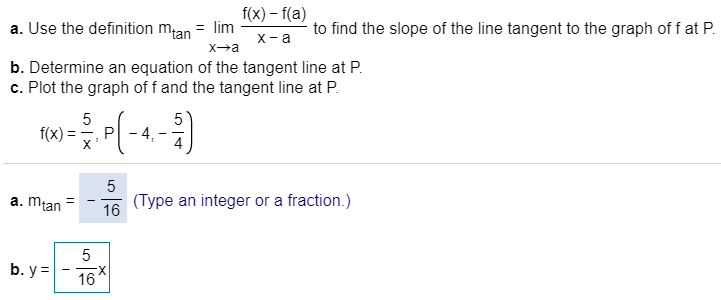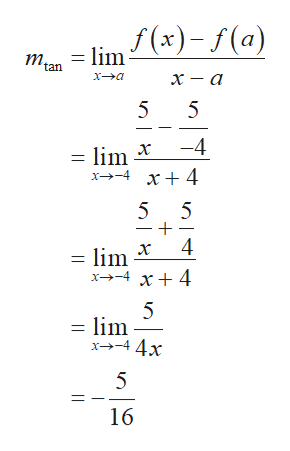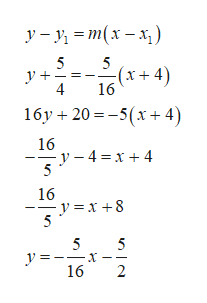f(x)-f(a)a. Use the definition mtan = limto find the slope of the line tangent to the graph of f at Pх -аX aXab. Determine an equation of the tangent line at Pc. Plot the graph of f and the tangent line at P55- 4,f(x)PX5(Type an integer or a fraction.)16a. mtan5b. y16LC

Questionhelp_outlineImage Transcriptionclosef(x)-f(a) a. Use the definition mtan = lim to find the slope of the line tangent to the graph of f at P х -а X a Xa b. Determine an equation of the tangent line at P c. Plot the graph of f and the tangent line at P 5 5 - 4, f(x) P X 5 (Type an integer or a fraction.) 16 a. mtan 5 b. y 16 LC fullscreen
Step 1
1. a) Obtain the slope of the line tangent to the graph of f at P,help_outlineImage Transcriptionclosef(x)-f(a) tan х — а х—а 5 5 -4 х = lim х—- 5 5 4 х =lim x4 x4 5 = lim x44x 5 16 fullscreen
Step 2

b) Obtain the equation of th...help_outlineImage Transcriptioncloseу -у - т(х - х,) 5 (x+4) 4 16 16y 205(x4 16 -4 x+4 5 16 y = x +8 5 5 5 y = 16 fullscreen

Want to see the full answer?

See Solution

Want to see this answer and more?

Our solutions are written by experts, many with advanced degrees, and available 24/7

See Solution
Tagged in

Other